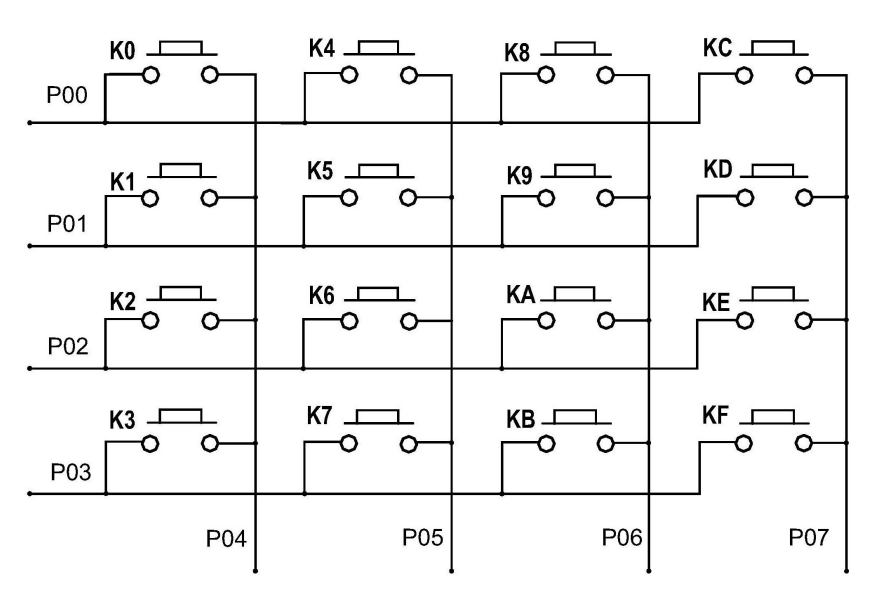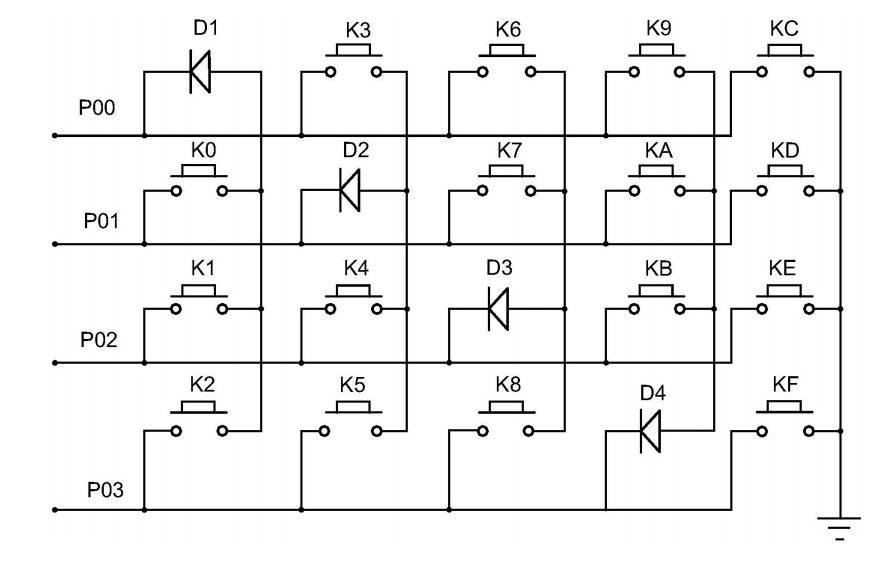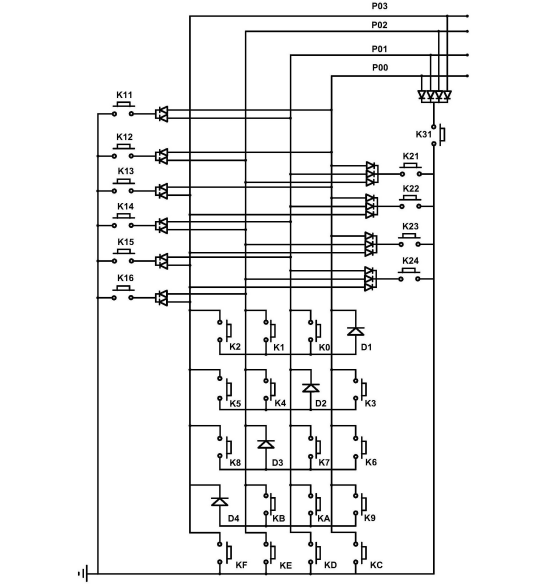# 矩阵键盘的改进(第一个专利)

4×5矩阵右侧的接地线；设置在第一列第一行、第二列第二行、第三列第三行、第四列第四行的电路单元为二极管，设置在4×5矩阵其余位置的电路单元为按键触点；4个二极管的正极与负极分别对应相应的列的导线和相应行的IO接口引线；设置在第一列、第二列、第三列、第四列的按键触点的一个引脚电连接相应列的导线，另一个引脚电连接相应行的IO接口引线，设置在第五列的按键触点的一个引脚电连接相应行的IO接口引线，另一个引脚电连接接地线。本发明使用4条IO接口引线实现 8 条接口引线完成的功能。main.c

  #include<reg51.h>

sbit  KEY0=P0^0;
sbit  KEY1=P0^1;
sbit  KEY2=P0^2;
sbit  KEY3=P0^3;
sbit  LED0=P1^0;
sbit  LED1=P1^1;
sbit  LED2=P1^2;
sbit  LED3=P1^3;
sbit  LED4=P1^4;
sbit  LED5=P1^5;
sbit  LED6=P1^6;
sbit  LED7=P1^7;
sbit  LED8=P3^0;
sbit  LED9=P3^1;
sbit  LED10=P3^2;
sbit  LED11=P3^3;
sbit  LED12=P3^4;
sbit  LED13=P3^5;
sbit  LED14=P3^6;
sbit  LED15=P3^7;

void   Key_Scan();
unsigned char key=0;

void main()
{
LED0=1; LED1=1;LED2=1;LED3=1;LED4=1;LED5=1;LED6=1;LED7=1;
LED8=1; LED9=1;LED10=1;LED11=1;LED12=1;LED13=1;LED14=1;LED15=1;
while(1)
{
Key_Scan();
switch(key)
{
case 1:LED0=~LED0;key=0;break;
case 2:LED1=~LED1;key=0;break;
case 3:LED2=~LED2;key=0;break;
case 4:LED3=~LED3;key=0;break;
case 5:LED4=~LED4;key=0;break;
case 6:LED5=~LED5;key=0;break;
case 7:LED6=~LED6;key=0;break;
case 8:LED7=~LED7;key=0;break;
case 9:LED8=~LED8;key=0;break;
case 10:LED9=~LED9;key=0;break;
case 11:LED10=~LED10;key=0;break;
case 12:LED11=~LED11;key=0;break;
case 13:LED12=~LED12;key=0;break;
case 14:LED13=~LED13;key=0;break;
case 15:LED14=~LED14;key=0;break;
case 16:LED15=~LED15;key=0;break;
default:break;
}
}
}

void  Key_Scan()   //按键扫描
{
unsigned int i;
if(((KEY0)&&(KEY1)&&(KEY2)&&(KEY3))!=1) //检测 1\2\3\4
{
for(i=200;i>0;i--);
if(((KEY0)&&(KEY1)&&(KEY2)&&(KEY3))!=1)
{
if(KEY0==0)  key=1;
if(KEY1==0)  key=2;
if(KEY2==0)  key=3;
if(KEY3==0)  key=4;
while(((KEY0)&&(KEY1)&&(KEY2)&&(KEY3))!=1);
for(i=200;i>0;i--);
}
}

if(((KEY0)&&(KEY1)&&(KEY2)&&(KEY3))==1)   //检测 5\6\7
{
for(i=2000;i>0;i--);
if(((KEY0)&&(KEY1)&&(KEY2)&&(KEY3))==1)
{
KEY0=0;							  //第一个IO赋值为0
if(((KEY1)&&(KEY2)&&(KEY3))!=1)
{
for(i=20;i>0;i--);
if(((KEY1)&&(KEY2)&&(KEY3))!=1)
{
if(KEY1==0) key=5;
if(KEY2==0) key=6;
if(KEY3==0) key=7;
while(((KEY1)&&(KEY2)&&(KEY3))!=1);
for(i=200;i>0;i--);
}
}
KEY0=1;
}
}

if(((KEY0)&&(KEY1)&&(KEY2)&&(KEY3))==1) 	//检测 8\9\10
{
for(i=2000;i>0;i--);
if(((KEY0)&&(KEY1)&&(KEY2)&&(KEY3))==1)
{
KEY1=0; 			    //第二个IO赋值为0
if(((KEY0)&&(KEY2)&&(KEY3))!=1)
{
for(i=20;i>0;i--);
if(((KEY0)&&(KEY2)&&(KEY3))!=1)
{
if(KEY0==0)     key=8;
if(KEY2==0)     key=9;
if(KEY3==0)     key=10;
while(((KEY0)&&(KEY2)&&(KEY3))!=1);
for(i=200;i>0;i--);
}
}
KEY1=1;
}
}

if(((KEY0)&&(KEY1)&&(KEY2)&&(KEY3))==1)	 	//检测 11\12 \13
{
for(i=2000;i>0;i--);
if(((KEY0)&&(KEY1)&&(KEY2)&&(KEY3))==1)
{
KEY2=0;  //第三个IO赋值为0
if(((KEY0)&&(KEY1)&&(KEY3))!=1)
{
for(i=20;i>0;i--);
if(((KEY0)&&(KEY1)&&(KEY3))!=1)
{
if(KEY0==0)     key=11;
if(KEY1==0)     key=12;
if(KEY3==0)     key=13;
while(((KEY0)&&(KEY1)&&(KEY3))!=1);
for(i=200;i>0;i--);
}
}
KEY2=1;
}
}

if(((KEY0)&&(KEY1)&&(KEY2)&&(KEY3))==1)	   //检测 14\15\16
{
for(i=2000;i>0;i--);
if(((KEY0)&&(KEY1)&&(KEY2)&&(KEY3))==1)
{
KEY3=0;	 //第四个IO赋值为0
if(((KEY0)&&(KEY1)&&(KEY2))!=1)
{
for(i=20;i>0;i--);
if(((KEY0)&&(KEY1)&&(KEY2))!=1)
{
if(KEY0==0)      key=14;
if(KEY1==0)      key=15;
if(KEY2==0)      key=16;
while(((KEY0)&&(KEY1)&&(KEY2))!=1);
for(i=200;i>0;i--);
}
}
KEY3=1;
}
}

}   

04-25517003-293787
04-221018
01-257250
11-103万+
12-094225
08-19547
06-23
11-0772
08-06
05-181万+
04-102009
01-052万+
05-275531
10-132万+
01-247591
10-102万+
12-272473
08-21